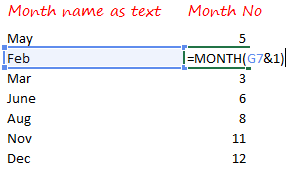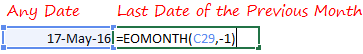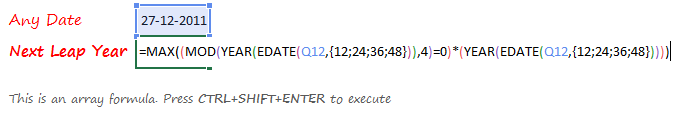If you often work with dates, you’ll find these hacks incredibility useful. Chances are that you might already know some of them and might find few new ones as well. Let’s jump right into it

## 1) Dates are Positive NumbersEvery once in a while dates act cocky and appear as random numbers. All you have got to do is convert them into a Date format using Ctrl + Shift + 3

Fun Fact 1 : Excel calendar begins from 1 Jan 1900. In any cell type 1 and convert that into a date format and take a look into the formula bar, you’ll see 1 Jan 1900. So the next time you a see a random number don’t freak out, it simply means the numbers of days past since 1 Jan 1900

Fun Fact 2 : You can convert the dates back to numbers using Ctrl + Shift + ~

## 2) Enter Today’s Date

There are 2 ways to do that

1. Use the shortcut Ctrl : (semicolon). The date will be static and will not change
2. Use the formula =TODAY() in any cell. The date will be dynamic and will change as per the calendar date

## 3) Find the no of days between 2 dates1. Subtract the smaller date from the larger date
2. One is added to include the starting date in the calculation (i.e. there are 10 days in between 1 Nov till 10 Nov)

## 4) Find the no of months between 2 dates1. The DATEDIF function can find the number of completed months between 2 dates.
2. This function is not documented in Excel, you can use it if you know it already 🙂

## 5) Find the no of years between 2 datesThere are a few more variation for using DATEDIF, you can learn all of them here

## 6) Find if the dates are between Start and End Dates1. The AND function is checking if the dates is between start and end
2. Learn how to use the IF AND Function

## 7) Display Month as Numbers1. The & operator combines the month name and 1 to form a date “1Feb”
2. The month function then extracts the month number from that date

I learnt this trick from Denys Calvin’s comment on Chandoo’s blog

## 8) Display week number from a date1. The WEEKNUM function returns the week number of any date
2. The first input is the date
3. The second input is the week start type, you can customize the day when your week starts## 9) Display the Day with the Date1. Select the date/dates
2. Press Ctrl 1 (to open format cells)
3. Go to Custom
4. Use the code : dddd dd-mmm-yy (4 times d, adds the day)

Fun Fact 1 : ddd adds the short form of Day for example Mon, Tue etc..

Fun Fact 2 : mmmm adds the full name of the month for example November, December etc..

Fun Fact 3 : mmmmm adds a initial letter of the month for example N (for November) D (for December) etc..

## 10) First Day of the Next MonthThe EOMONTH returns a month ending date. It accepts 2 inputs

1. The Start Date – Could be any date
2. Months – The numbers of months you want to go forward or backward. Use – minus sign to go backwards

Plus 1 shifts the month ending date to the start of the next month

## 11) First Day of the Current MonthThe logic and formula remains the same just the month number in the EOMONTH becomes -1

## 12) Last Day of the Current MonthSimple EOMONTH with months as 0 will work 🙂

## 13) Last Day of the Previous MonthThe month input becomes -1 to get the last date of the previous month

## 14) Get Weekdays Only1. Simple IF Formula Checks if the previous day is Friday (i.e. weekday = 5)
2. If yes then it jumps to Monday (previous day + 3)
3. Else jumps by a single day (previous day + 1)

## 15) Show Alternate Dates1. Write the first 3 alternate dates
2. Drag the cells down, selecting the first 3 cells and alternate dates will be auto filled

## 16) Find the next leap year1. The formula is checking which of the next 4 years are divisible by 4.
2. If yes then the formula picks up that year

Fun Fact :

1. This formula will always give the next leap year even if the current date belongs to a leap year
2. If you want the current leap year also to be considered tweak the formula like this

=MAX((MOD(YEAR(EDATE(Q12,{0;12;24;36})),4)=0)*(YEAR(EDATE(Q12,{0;12;24;36}))))

Just be sure to press CTRL SHIFT ENTER to confirm the formula

## 17) Find the next SundayTo customize this formula to any other day, just change = 7 to any other week number. 1= Monday and 7 = Sunday

## What are your Date Tips, Tricks and Hacks

If you have a favorite tip on dates or have found any of these useful, please share using comments